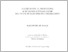# Mathematical modelling & EP based approach for sea wave in electricity generation / Baharin Abu Bakar

Abu Bakar, Baharin (2014) Mathematical modelling & EP based approach for sea wave in electricity generation / Baharin Abu Bakar. Masters thesis, Universiti Teknologi MARA.

## Abstract

Generally, energy is important to enhance countries development. Electricity demand is increasing drastically in the last few decades. Fossil fuel which is considered a common source and utilized globally has shown reduction due to the progressing energy demand. Most governments in the developing countries are giving incentives and funds to the researchers to produce energy based on renewable energy resources. This has been identified as free from environmental threats. Many efforts have been conducted worldwide to promote electricity based on renewable energy resources. European countries, Japan, USA and India are the countries which have continuously conducted research; in particular, the energy from the sea waves. The main objective of this study is to innovate new design of electricity generator using sea wave as the energy source. In order to achieve this, mathematical modeling is initially developed which represents all the related mathematical equation in the system. This thesis presents the mathematical modeling and Evolutionary Programming (EP) approach of sea wave energy for electricity generation. The study begins with the derivations of mathematical equations for each component in the electricity generation system taking the sea wave as the input of the whole system. The developed mathematical equations for each stage in the system verified, while validation is conducted in order to realize its workability. Electrical and mechanical relationships are derived to relate the workability of each component in the system for the purpose of electricity generation. Various experiments have been conducted to realize the variation of results for chosen modules which eventually can lead to the generation of electricity. Artificial Intelligence (AI) approach namely Evolutionary Programming (EP) is implemented to compute the optimal parameters for the developed model. Results obtained from the experiment indicated that the proposed mathematical modeling is feasible to generate electricity by using the sea wave energy.Preview
Text
TM_BAHARIN ABU BAKAR EE 14_5.pdf

Item Type: Thesis (Masters)
Creators:
CreatorsID Num.
Abu Bakar, BaharinUNSPECIFIED
Divisions: Faculty of Electrical Engineering
Item ID: 17373
Uncontrolled Keywords: Mathematical modelling; EP based approach; Sea wave; Electricity generation
URI: http://ir.uitm.edu.my/id/eprint/17373View Item